## 6.2.14 LocalizeMUSIC

### 6.2.14.1 Outline of the node

From multichannel speech waveform data, direction-of-arrival (DOA) in the horizontal plane is estimated using the MUltiple SIgnal Classification (MUSIC) method. It is the main node for Sound Source Localization in HARK  .

### 6.2.14.2 Necessary file

The transfer function file which consists of a steering vector is required. It is generated based on the positional relationship between the microphone and sound, or the transfer function for which measurement was performed.

### 6.2.14.3 Usage

This node estimates a sound’s direction and amount of power using the MUSIC method. Detection of a direction with high power in each frame allows the system to know the direction of sound, the number of sound sources, the speech periods, etc. to some extent. The orientation result outputted from this node is used for post-processing such as tracking and source separation.

Typical connection

Figure 6.29 shows a typical connection example.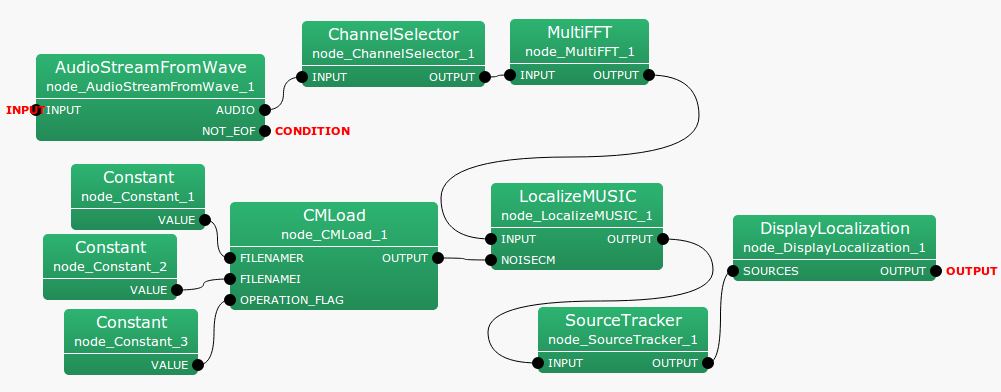Figure 6.29: Connection example of LocalizeMUSIC

### 6.2.14.4 Input-output and property of the node

Input

INPUT

: Matrix<complex<float> > , Complex frequency representation of input signals with size $M \times (NFFT/2+1)$.

NOISECM

: Matrix<complex<float> >  type. The correlation matrix for each frequency bin. The $NFFT/2 + 1$ correlation matrices are inputted, corresponding to the $M$-th complex square matrix. The rows of Matrix<complex<float> >  express frequency ($NFFT / 2+1$ rows) and the columns express the complex correlation matrix ($M * M$ columns). This input terminal can also be left disconnected; then an identity matrix is used for the correlation matrix instead.

Output

OUTPUT

: Source position (direction) is expressed as Vector<ObjectRef>  type. ObjectRef  is a Source  and is a structure which consists of the power of the MUSIC spectrum of the source and its direction. The element number of Vector  is a sound number ($N$). Please refer to node details for the details of the MUSIC spectrum.

SPECTRUM

: Vector<float>  type. Power of the MUSIC spectrum for every direction. The output is equivalent to $\bar{P}(\theta )$ in Eq. (). In case of three dimensional sound source localization, $\theta$ is a three dimensional vector, and $\bar{P}(\theta )$ becomes three dimensional data. Please refer to node details for the detail of the output format. This output terminal is not displayed by default.

Refer to Figure 6.30 for the addition method of hidden output.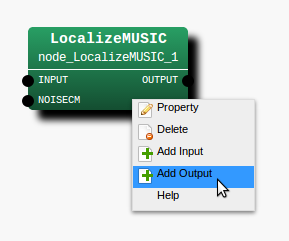Step 1: Right-click LocalizeMUSIC  and click Add Output.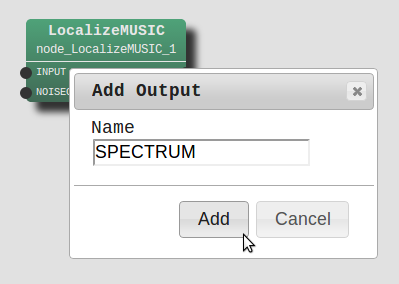Step 2: Enter SPECTRUM in the input, then, click Add.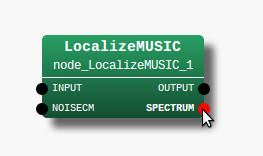Step 3: The SPECTRUM output terminal is added to the node.
Figure 6.30: Usage example of hidden outputs : Display of SPECTRUM terminal
Table 6.28: Parameter list of LocalizeMUSIC
 Parameter name Type Default value Unit description MUSIC_ALGORITHM SEVD Algorithm of MUSIC TF_CHANNEL_SELECTION See below. Channel number used LENGTH 512 [pt] FFT points ($NFFT$) SAMPLING_RATE 16000 [Hz] Sampling rate A_MATRIX Transfer function file name WINDOW 50 [frame] Frames to normalize CM WINDOW_TYPE FUTURE Frame selection to normalize CM PERIOD 50 [frame] The cycle to compute SSL NUM_SOURCE 2 Number of sounds MIN_DEG -180 [deg] Minimum azimuth MAX_DEG 180 [deg] Maximum azimuth LOWER_BOUND_FREQUENCY 500 [Hz] Lower bound frequency UPPER_BOUND_FREQUENCY 2800 [Hz] Upper bound frequency SPECTRUM_WEIGHT_TYPE Uniform Type of frequency weight A_CHAR_SCALING 1.0 Coefficient of weight MANUAL_WEIGHT_SPLINE See below. Coefficient of spline weight MANUAL_WEIGHT_SQUARE See below. Key point of rectangular weight ENABLE_EIGENVALUE_WEIGHT true Enable eigenvalue weight ENABLE_INTERPOLATION false Enable interpolation of TFs INTERPOLATION_TYPE FTDLI Selection of TF interpolation HEIGHT_RESOLUTION 1.0 [deg] Interval of elevation AZIMUTH_RESOLUTION 1.0 [deg] Interval of azimuth RANGE_RESOLUTION 1.0 [m] Interval of radius PEAK_SEARCH_ALGORITHM LOCAL_MAXIMUM Peak search algorithm MAXNUM_OUT_PEAKS -1 Max. num. of output peaks DEBUG false ON/OFF of debug output

Parameter

MUSIC_ALGORITHM

: string  type. Selection of algorithm used in order to calculate the signal subspace in the MUSIC method. SEVD represents standard eigenvalue decomposition, GEVD represents generalized eigenvalue decomposition, and GSVD represents generalized singular value decomposition. LocalizeMUSIC  enters a correlation matrix with sound information from the NOISECM terminal, and possesses a function which can do SSL whitening of the noise (suppression). SEVD realizes SSL without the function. When SEVD is chosen, the input from NOISECM terminal is disregarded. Although both GEVD and GSVD have a function to whiten the noise inputted from the NOISECM terminal, GEVD has better noise suppression performance compared with GSVD. It has the problem that the calculation time takes approximately 4 times longer. Depending on the scene and computing environment, you can select one of the three algorithms. Please refer to node details for the details of algorithm.

TF_CHANNEL_SELECTION

: Vector<int>  type. Of steering vectors of multichannel stored in the transfer function file, it is parameters which chooses the steering vector of specified channel to use. The channel number begins from 0 like ChannelSelector . Signal processing of 8 channel is assumed by default and it is set as <Vector<int> 0 1 2 3 4 5 6 7> . It is necessary to align the number ($M$) of elements of the parameters with the channel number of incoming signals. Moreover, it is necessary to align the order of channel and the channel order of TF_CHANNEL_SELECTION to be inputted into INPUT terminal.

LENGTH

: int  type. 512 is the default value. FFT point in the case of fourier transform. It is necessary to align it with the FFT points to the preceding paragraph.

SAMPLING_RATE

: int  type. 16000 is the default value. Sampling frequency of input acoustic signal. It is necessary to align with other nodes like LENGTH.

A_MATRIX

: string type. There is no default value. The file name of the transfer function file is designated. Both absolute path and relative path are supported. Refer to the harktool4 for the creation method of the transfer function file.

WINDOW

: int  type. 50 is the default value. The number of smoothing frames for correlation matrix calculation is designated. Within the node, the correlation matrix is generated for every frame from the complex spectrum of the input signal, and the addition mean is taken by the number of frames specified in WINDOW. Although the correlation matrix will be stabilized if this value is enlarged, time delays become long due to the long interval.

WINDOW_TYPE

: string  type. FUTURE is the default value. The selection of used smoothing frames for correlation matrix calculation. Let $f$ be the current frame. If FUTURE, frames from $f$ to $f+WINDOW-1$ will be used for the normalization. If MIDDLW, frames from $f-(WINDOW/2)$ to $f+(WINDOW/2)+(WINDOW\% 2)-1$ will be used for the normalization. If PAST, frames from $f-WINDOW+1$ to $f$ will be used for the normalization.

PERIOD

: int  type. 50 is the default value. The cycle of SSL calculation is specified in frames number. If this value is large, the time interval for obtaining the orientation result becomes large, which will result in improper acquisition of the speech interval or bad tracking of the mobile sound. However, since the computational cost increases if it is small, tuning according to the computing environment is needed.

NUM_SOURCE

: int  type. 2 is the default value. It is the number of dimensions of the signal subspace in the MUSIC method, and can be practically interpreted as the number of desired sound sources to be emphasized in the peak detection. It is expressed as $N_ s$ in the following nodes details. It should be $1 \leq N_ s \leq M - 1$. It is desirable to match the sound number of the desired sound, but, for example, in the case of the number of desired sound sources being 3, the interval that each sound pronounces is different, thus, it is sufficient to select a smaller value than it is practically.

MIN_DEG

: int  type. -180 is the default value. It is the minimum angle for peak search, and is expressed as $\theta _{min}$ in the node details. 0 degree is the robot front direction, negative values are the robot right hand direction, and positive values are the robot left-hand direction. Although the specified range is considered as $\pm 180$ degrees for convenience, since the surrounding range of 360 degrees or more is also supported, there is no particular limitation.

MAX_DEG

: int  type. 180 is the default value. It is the maximum angle for peak search, and is expressed as $\theta _{max}$ in the node details. Others are the same as that of MIN_DEG.

LOWER_BOUND_FREQUENCY

: int  type. 500 is the default value. It is the minimum of frequency bands which is taken into consideration for peak detection, and is expressed as $\omega _{min}$ in the node details. It should be $0 \leq \omega _{min} \leq {\rm SAMPLING\_ RATE} / 2$.

UPPER_BOUND_FREQUENCY

: int  type. 2800 is the default value. It is the maximum of frequency bands Which is taken into consideration for peak detections, and, is expressed as $\omega _{max}$ below. It should be $\omega _{min} < \omega _{max} \leq {\rm SAMPLING\_ RATE} / 2$.

SPECTRUM_WEIGHT_TYPE

: string  type. ‘Uniform’ is the default value. The distribution of weights against the frequency axial direction of the MUSIC spectrum used for peak detections is designated. ‘Uniform’ sets weights to OFF. ‘A_Characteristic’ gives the MUSIC spectrum weights imitating the sound pressure sensitivity of human hearing. ‘Manual_Spline’ gives the MUSIC spectrum weights suited to the Cubic spline curve for which the point specified in MANUAL_WEIGHT_SPLINE is considered as the interpolating point. ‘Manual_Square’ generates the rectangular weights suited to the frequency specified in MANUAL_WEIGHT_SQUARE, and gives it to MUSIC spectrum.

A_CHAR_SCALING

: float  type. 1.0 is the default value. This is scaling term which modifies the A characteristic weight on the frequency axis. Since the A characteristic weight imitates the sound pressure sensitivity of human’s hearing, filtering to suppress sound outside of the speech frequency range is possible. Although the A characteristic weight has a standard, depending on the general sound environment, noise may enter the speech frequency range, and it may be unable to orientate well. Then, the A characteristic weight should be increased, causing more suppression, especially in the lower frequencies.

MANUAL_WEIGHT_SPLINE

: Matrix<float>  type.
<Matrix<float> <rows 2> <cols 5> <data 0.0 2000.0 4000.0 6000.0 8000.0 1.0 1.0 1.0 1.0 1.0> > is the default value. It is designated with the float value 2-by-$K$ matrix. $K$ is equivalent to the number of interpolation points for spline interpolations. The first row specifies the frequency and the second row specifies the weight corresponding to it. Weighting is performed according to the spline curve which passes along the interpolated point. By default, the weights are all set to 1 for the frequency bands from 0 [Hz] to 8000 [Hz] .

MANUAL_WEIGHT_SQUARE

: Vector<float>  type. <Vector<float> 0.0 2000.0 4000.0 6000.0 8000.0> is the default value. By the frequency specified in MANUAL_WEIGHT_SQUARE, the rectangular weight is generated and is given to MUSIC spectrum. For the frequency bands from the odd components of MANUAL_WEIGHT_SQUARE to the even components, the weight of 1 is given, and for the frequency bands from the even components to the odd components, the weight of 0 is given. By default, the MUSIC spectrum from 2000 [Hz] to 4000 [Hz] and 6000 [Hz] to 8000 [Hz] can be suppressed.

ENABLE_EIGENVALUE_WEIGHT

: bool  type. True is the default value. For true, in the case of calculation of the MUSIC spectrum, the weight is given as the square root of the maximum eigenvalue (or the maximum singular value) acquired from eigenvalue decomposition (or singular value decompositions) of the correlation matrix. Since this weight greatly changes depending on the eigenvalue of the correlation matrix inputted from NOISECM terminal when choosing GEVD and GSVD for MUSIC_ALGORITHM, it is good to choose false.

ENABLE_INTERPOLATION

: bool  type. False is the default value. In case of true, the spatial resolution of sound source localization can be improved by the interpolation of transfer functions specified by A_MATRIX. INTERPOLATION_TYPE specifies the method for the interpolation. The new resolution after the interpolation can be specified by HEIGHT_RESOLUTION, AZIMUTH_RESOLUTION, and RANGE_RESOLUTION, respectively.

INTERPOLATION_TYPE

: string  type. FTDLI is the default value. This specifies the interpolation method for transfer functions.

HEIGHT_RESOLUTION

: float  type. 1.0[deg] is the default value. This specifies the interval of elevation for the transfer function interpolation.

AZIMUTH_RESOLUTION

: float  type. 1.0[deg] is the default value. This specifies the interval of azimuth for the transfer function interpolation.

RANGE_RESOLUTION

: float  type. 1.0[m] is the default value. This specifies the interval of radius for the transfer function interpolation.

PEAK_SEARCH_ALGORITHM

: string type. LOCAL_MAXIMUM is the default. This selects the algorithm for searching peaks from the MUSIC spectrum. If LOCAL_MAXIMUM, peaks are defined as the maximum point among all adjacent points (local maximum). If HILL_CLIMBING, peaks are firstly searched only on the horizontal plane (azimuth search) and then searched in the vertical plane of detected azimuth (elevation search).

MAXNUM_OUT_PEAKS

: int type. -1 is the default. This parameter defines the maximum number of output peaks of MUSIC spectrum (sound sources). If 0, all the peaks are output. If MAXNUM_OUT_PEAKS $> 0$, MAXNUM_OUT_PEAKS peaks are output in order of their power. If -1, MAXNUM_OUT_PEAKS = NUM_SOURCE.

DEBUG

: bool  type. ON/OFF of the debug output and the format of the debug output are as follows. First, the set of index of sound, direction, and power is outputted in tab delimited for only several number of sound detected in frames. ID is the number given for convenience in order from 0 for every frame, though the number itself is meaningless. For direction [deg], an integer with rounded decimal is displayed. As for power, the power value of MUSIC spectrum $\bar{P}(\theta )$ of Eq. () is outputted as is. Next, “MUSIC spectrum:” is outputted after a line feed, and the value of $\bar{P}(\theta )$ of Eq. () is displayed for all $\theta$.

### 6.2.14.5 Details of the node

The MUSIC method is the method of estimating the direction-of-arrival (DOA) utilizing the eigenvalue decomposition of the correlation matrix among input signal channels. The algorithm is summarized below.

Generation of transfer function :

In the MUSIC method, the transfer function from sound to each microphone is measured or calculated numerically and it is used as a priori information. If the transfer function in the frequency domain from sound $S(\theta )$ in direction $\theta$ in view of microphone array to the $i$-th microphone $M_ i$ is set to $h_ i(\theta ,\omega )$, the multichannel transfer function multichannel can be expressed as follows.

 \begin{equation} \label{eq:tf} {\boldsymbol H}(\theta ,\omega ) = [h_1(\theta ,\omega ),\cdots ,h_ M(\theta ,\omega )] \end{equation} (8)

This transfer function vector is prepared for every suitable interval delta, $\Delta \theta$ (non-regular intervals are also possible) by calculation or measurement in advance. In HARK  , harktool4 is offered as a tool which can generate the transfer function file also by numerical calculation and also by measurement. Please refer to the paragraph of harktool4 for the prepare a specific transfer function file (From harktool4, we can create the database of three dimensional transfer functions for three dimensional sound source localization). In the LocalizeMUSIC  node, this a priori information file (transfer function file) is imported and used with the file name specified in A_MATRIX. Thus, since the transfer function is prepared for every direction of sound and is scanned to the direction using the direction vector (or transfer function, in the case of orientation), it is sometimes called ‘steering vector’.

Calculation of correlation matrix between the inputs signal channels :

The operation by HARK   begins from here. First, the signal vector in the frequency domain obtained by short-time fourier transform of the input acoustic signal in $M$ channel is found as follows.

 \begin{equation} {\boldsymbol X}(\omega ,f) = [X_1(\omega ,f), X_2(\omega ,f), X_3(\omega ,f), \cdots , X_ M(\omega ,f)]^ T, \label{eq:LocalizeMUSIC_ cor} \end{equation} (9)

where $\omega$ expresses frequency and $f$ expresses frame index. In HARK  , the process so far is performed by the MultiFFT  node in the preceding paragraph.

The correlation matrix between channels of the incoming signal ${\boldsymbol X}(\omega ,f)$ can be defined as follows for every frame and for every frequency .

 \begin{equation} {\boldsymbol R}(\omega ,f) = {\boldsymbol X}(\omega ,f){\boldsymbol X}^*(\omega ,f) \label{eq:LocalizeMUSIC_1} \end{equation} (10)

where $()^*$ represents the conjugate transpose operator. If this ${\boldsymbol R}(\omega ,f)$ is utilized in following processing as is, theoretically, it will be satisfactory, but practically, in order to obtain the stable correlation matrix, those time averaging is used in HARK  .

 \begin{equation} {\boldsymbol R}’(\omega ,f) = \frac{1}{{\rm WINDOW}}\sum _{i=W_ i}^{W_ f}{\boldsymbol R}(\omega ,f+i) \label{eq:LocalizeMUSIC_ period} \end{equation} (11)

The frames used for the averaging can be changed by WINDOW_TYPE. If WINDOW_TYPE=FUTURE, $W_ i = 0$ and $W_ f = {\rm WINDOW}-1$. If WINDOW_TYPE=MIDDLE, $W_ i = -{\rm WINDOW}/2$ and $W_ f = {\rm WINDOW}/2+{\rm WINDOW}\% 2-1$. If WINDOW_TYPE=PAST, $W_ i = -{\rm WINDOW}+1$ and $W_ f = 0$.

Decomposition to the signal and noise subspace :

In the MUSIC method, an eigenvalue decomposition or singular value decomposition of the correlation matrix ${\boldsymbol R}’(\omega ,f)$ found in the Eq. () is performed and the $M$-th space is decomposed into the signal subspace and the other subspace.

Since the processing has high computational cost, it is designed to be calculated only once in several frames. In LocalizeMUSIC , this operation period can be specified in PERIOD.

In LocalizeMUSIC , the method for decomposing into subspace can be specified by MUSIC_ALGORITHM.

When MUSIC_ALGORITHM is specified for SEVD, the following standard eigenvalue decomposition is performed.

 \begin{equation} {\boldsymbol R}’(\omega ,f) = {\boldsymbol E}(\omega ,f) {\boldsymbol \Lambda }(\omega ,f) {\boldsymbol E}^{-1}(\omega ,f)~ , \label{eq:LocalizeMUSIC_ SEVD} \end{equation} (12)

where ${\boldsymbol E}(\omega ,f)$ represents the matrix ${\boldsymbol E}(\omega ,f) = [{\boldsymbol e}_1(\omega ,f), {\boldsymbol e}_2(\omega ,f), \cdots , {\boldsymbol e}_ M(\omega ,f)]$ which consists of singular vectors which perpendicularly intersect each other, and ${\boldsymbol \Lambda }(\omega )$ represents the diagonals matrix using the eigenvalue corresponding to individual eigenvectors as the diagonal component. In addition, the diagonal component of ${\boldsymbol \Lambda }(\omega )$, $[\lambda _1(\omega ), \lambda _2(\omega ),\dots ,\lambda _ M(\omega )]$ is considered to have been sorted in descending order.

When MUSIC_ALGORITHM is specified for GEVD, the following generalized eigenvalue decomposition is performed.

 \begin{equation} {\boldsymbol K}^{-\frac{1}{2}}(\omega ,f){\boldsymbol R}’(\omega ,f){\boldsymbol K}^{-\frac{1}{2}}(\omega ,f) = {\boldsymbol E}(\omega ,f) {\boldsymbol \Lambda }(\omega ,f) {\boldsymbol E}^{-1}(\omega ,f)~ , \label{eq:LocalizeMUSIC_ GEVD} \end{equation} (13)

where ${\boldsymbol K}(\omega ,f)$ represents the correlation matrix inputted from NOISECM terminal at the $f$-th frame. Since large eigenvalues from the noise sources included in ${\boldsymbol K}(\omega ,f)$ can be whitened (surpressed) using generalized eigenvalue decomposition with ${\boldsymbol K}(\omega ,f)$, SSL with suppressed noise is realizable.

When MUSIC_ALGORITHM is specified for GSVD, the following generalized singular value decomposition is performed.

 \begin{equation} {\boldsymbol K}^{-1}(\omega ,f){\boldsymbol R}’(\omega ,f) = {\boldsymbol E}(\omega ,f) {\boldsymbol \Lambda }(\omega ,f) {\boldsymbol E}_ r^{-1}(\omega ,f)~ , \label{eq:LocalizeMUSIC_ GSVD} \end{equation} (14)

where ${\boldsymbol E}(\omega ,f), {\boldsymbol E}_ r(\omega ,f)$ represents the matrix which consists of left singular vector and right singular vector, respectively, and ${\boldsymbol \Lambda }(\omega )$ represents the diagonal matrix using each singular-value as the diagonal components.

Since the eigenvalue (or singular-value) corresponding to eigen vector space ${\boldsymbol E}(\omega ,f)$ obtained by degradation has correlation with the power of sound, by taking eigen vector corresponding to the eigenvalue with the large value, only the subspace of loud desired sound with large power can be chosen. If the number of sounds to be considered is set to $N_ s$, then eigen vector $[e_1(\omega ), \cdots , e_{N_ s}(\omega )]$ corresponds to the sound, are eigen vector $[e_{N_ s+1}(\omega ), \cdots , e_ M(\omega )]$ corresponds to noise. In LocalizeMUSIC , $N_ s$ can be specified as NUM_SOURCE.

Calculation of MUSIC spectrum :

The MUSIC spectrum for SSL is calculated as follows using only noise-related eigen vectors.

 \begin{equation} P(\theta ,\omega ,f) = \frac{\left| {\boldsymbol H}^*(\theta ,\omega ) {\boldsymbol H}(\theta ,\omega ) \right|}{\sum _{i=N_ s+1}^ M \left| {\boldsymbol H}^*(\theta ,\omega ) e_ i(\omega ,f) \right|} \label{eq:LocalizeMUSIC_ music_ spectrum_ bin} \end{equation} (15)

In the denominator in the right-hand side, the inner product of the noise-related eigen vector and steering vector is calculated. On the space spanned by the eigen vector, since the noise subspace corresponding to small eigenvalue and the signal subspace corresponding to a large eigenvalue intersect perpendicularly each other, if the transfer function is a vector corresponding to the desired sound, this inner product will be 0 theoretically. Therefore, $P(\theta ,\omega ,f)$ diverges infinitely. In fact, although it does not diverge infinitely under the effect of noise etc., a sharp peak is observed compared to beam forming. The right-hand side of the numerator is an normalization term.

Since $P(\theta ,\omega ,f)$ is MUSIC spectrum obtained for every frequency, broadband SSL is performed as follows.

 \begin{equation} \bar{P}(\theta ,f) = \sum _{\omega =\omega _{min}}^{\omega _{max}} W_{\Lambda }(\omega ,f) W_{\omega }(\omega ,f) P(\theta ,\omega ,f)~ , \label{eq:LocalizeMUSIC_ music_ spectrum} \end{equation} (16)

where $\omega _{min}$ and $\omega _{max}$ show the minimum and maximum of the frequency bands which are handled in the broadband integration of MUSIC spectrum, respectively, and they can be specified as LOWER_BOUND_FREQUENCY and UPPER_BOUND_FREQUENCY in LocalizeMUSIC , respectively.

Moreover, $W_{\Lambda }(\omega ,f)$ is the eigen-value weight in the case of broadband integration and is square root of the maximum eigenvalue (or maximum singular-value).

In LocalizeMUSIC , the presence or absence of eigenvalue weight can be chosen by ENABLE_EIGENVALUE_WEIGHT, and in case of false, it is $W_{\Lambda }(\omega ,f) = 1$ and in case of true, it is $W_{\Lambda }(\omega ,f) = \sqrt {\lambda _1(\omega ,f)}$. Moreover, $W_{\omega }(\omega ,f)$ is the frequency weight in the case of broadband integration, and the type can be specified as follows by SPECTRUM_WEIGHT_TYPE in LocalizeMUSIC .

• In the case that SPECTRUM_WEIGHT_TYPE is Uniform
weights become uniform and $W_{\omega }(\omega ,f) = 1$ all frequency bins.

• In the case that SPECTRUM_WEIGHT_TYPE is A_Characteristic
it will be A characteristic weight $\mathcal{W}(\omega )$ which the International Electrotechnical Commission standardizes. The frequency characteristics of A characteristic weight is shown in Figure 6.31. The horizontal axis is $\omega$ and the vertical axis is $\mathcal{W}(\omega )$. In LocalizeMUSIC , the scaling term A_CHAR_SCALING of frequency direction is introduced to the frequency characteristic. If A_CHAR_SCALING is set as $\alpha$, then the frequency weight actually used can be expressed as $\mathcal{W}(\alpha \omega )$. In Figure 6.31, the case of $\alpha = 1$ and the case of $\alpha = 4$ are plotted as an example. The weight finally applied to the MUSIC spectrum is $W_{\omega }(\omega ,f) = 10^{\frac{\mathcal{W}(\alpha \omega )}{20}}$. As an example, $W_{\omega }(\omega ,f)$ when A_CHAR_SCALING=1 is shown in Figure 6.32.

• When SPECTRUM_WEIGHT_TYPE is Manual_Spline
it is the frequency weight in line with the curve in which the spline interpolation was carried out for the interpolating point specified in MANUAL_WEIGHT_SPLINE. MANUAL_WEIGHT_SPLINE is specified with the Matrix<float>  type of 2-by-$k$ matrix. The first row represents the frequency and the second row represents the weight for the frequency. The interpolation mark $k$ may be any point. As an example, in the case that MANUAL_WEIGHT_SPLINE is
<Matrix<float> <rows 2> <cols 3> <data 0.0 4000.0 8000.0 1.0 0.5 1.0> >
the number of interpolation points is 3, and the spline curve to which the weight of 1, 0.5, and 1 is applied in three frequencies, 0, 4000, and 8000[Hz], on the frequency axis, respectively can be created. $W_{\omega }(\omega ,f)$ at that time is shown in Figure 6.33.

• When SPECTRUM_WEIGHT_TYPE is Manual_Square
it is the frequency weight in line with the rectangular weight from which the rectangle changes at the frequency specified in MANUAL_WEIGHT_SQUARE. MANUAL_WEIGHT_SQUARE is specified in the $k$-th Vector<float>  type, and expresses the frequency to switch the rectangle. The number $k$ of the switching point is arbitrary. As an example, the rectangle weight $W_{\omega }(\omega ,f)$ in the case that MANUAL_WEIGHT_SQUARE is considered as
<Vector<float> 0.0 2000.0 4000.0 6000.0 8000.0>
is shown in Figure 6.34. By using this weight, two or more frequency domains which cannot be specified with only UPPER_BOUND_FREQUENCY and LOWER_BOUND_FREQUENCY can be chosen.

The output port SPECTRUM outputs the result of $\bar{P}(\theta ,f)$ in Eq. () as a one dimensional vector. In case of three dimensional sound source localization, $\bar{P}(\theta ,f)$ becomes three dimensional data, and $\bar{P}(\theta ,f)$ is converted to one dimensional vector and output from the port. Let Ne, Nd, and Nr denote the number of elevation, the number of azimuth, and the number of radius, respectively. Then, the conversion is described as follows.

FOR ie = 1 to Ne
FOR id = 1 to Nd
FOR ir = 1 to Nr
SPECTRUM[ir + id * Nr + ie * Nr * Nd] = P[ir][id][ie]
ENDFOR
ENDFOR
ENDFOR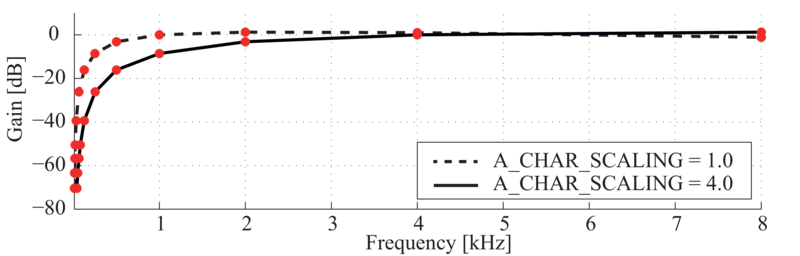Figure 6.31: Frequency characteristic of characteristic weight when considering as SPECTRUM_WEIGHT_TYPE=A_Characteristic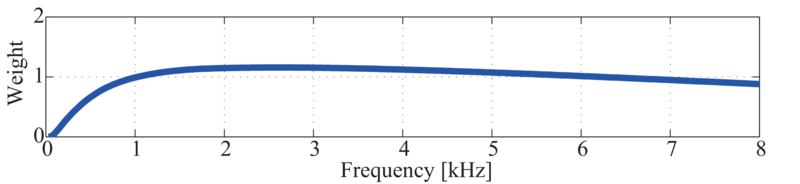Figure 6.32: $W_{\omega }(\omega ,f)$ in case of SPECTRUM_WEIGHT_TYPE=A_Charasteristic and A_CHAR_SCALING=1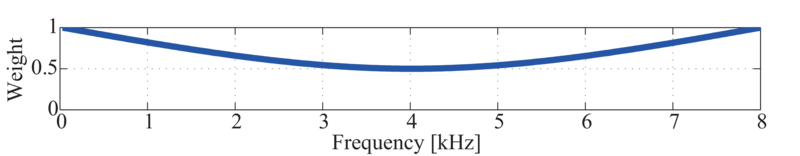Figure 6.33: $W_{\omega }(\omega ,f)$ in case of SPECTRUM_WEIGHT_TYPE=Manual Spline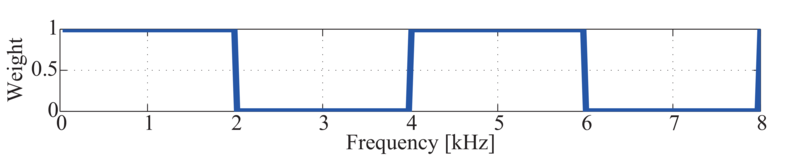Figure 6.34: $W_{\omega }(\omega ,f)$ in case of SPECTRUM_WEIGHT_TYPE=Manual_Square

Search of sound :

Next, the peak is detected from the range in $\theta _{min}$ to $\theta _{max}$ for $\bar{P}(\theta ,f)$ of Eq. (), and the power of the MUSIC spectrum corresponding to DoA for the top MAXNUM_OUT_PEAKS are outputted in descending order of the value. Moreover, the number of output sound sources may become below when the number of peaks does not reach to MAXNUM_OUT_PEAKS. The algorithm for searching peaks can be selected by PEAK_SEARCH_ALGORITHM whether it is the local maximum searching or the hill-climbing method. In LocalizeMUSIC , $\theta _{min}$ and $\theta _{max}$ of azimuth can be specified in MIN_DEG and MAX_DEG, respectively. The module uses all elevation and radius for the sound source search.

Discussion :

Finally, we describe the effect that whitening (noise suppression0 has on MUSIC spectrum in Eq. () when choosing GEVD and GSVD for MUSIC_ALGORITHM.

Here, as an example, consider the situation of four speakers (Directions = 75[deg], 25[deg], -25[deg], and -75[deg]) speaking simultaneously.

Figure 6.35(a) shows the result of choosing SEVD for MUSIC_ALGORITHM and not having whitened the noise. The horizontal axis is the azimuth, the vertical axis is frequency, and the value is $P(\theta ,\omega ,f)$ of the Eq. (). As shown in the figure, there is diffusion noise in the low frequency domain and -150 degree direction, which reveals that the peak is not correctly detectable to only the direction of the 4 speakers.

Figure 6.35(b) shows the MUSIC spectrum in the interval in which SEVD is chosen for MUSIC_ALGORITHM and 4 speakers do not perform speech. The diffusion noise and the direction noise observed can be seen in Figure 6.35(a).

Figure 6.35(c) is the MUSIC spectrum when generating ${\boldsymbol K}(\omega ,f)$ from the information on Figure 6.35(b), choosing GSVD for MUSIC_ALGORITHM as general sound information, and whitening the noise. As shown in the figure, it can be seen that the diffusion noise and the direction noise contained in ${\boldsymbol K}(\omega ,f)$ are suppressed correctly and the strong peaks are only in the direction of the 4 speakers.

Thus, it is useful to use GEVD and GSVD for known noise.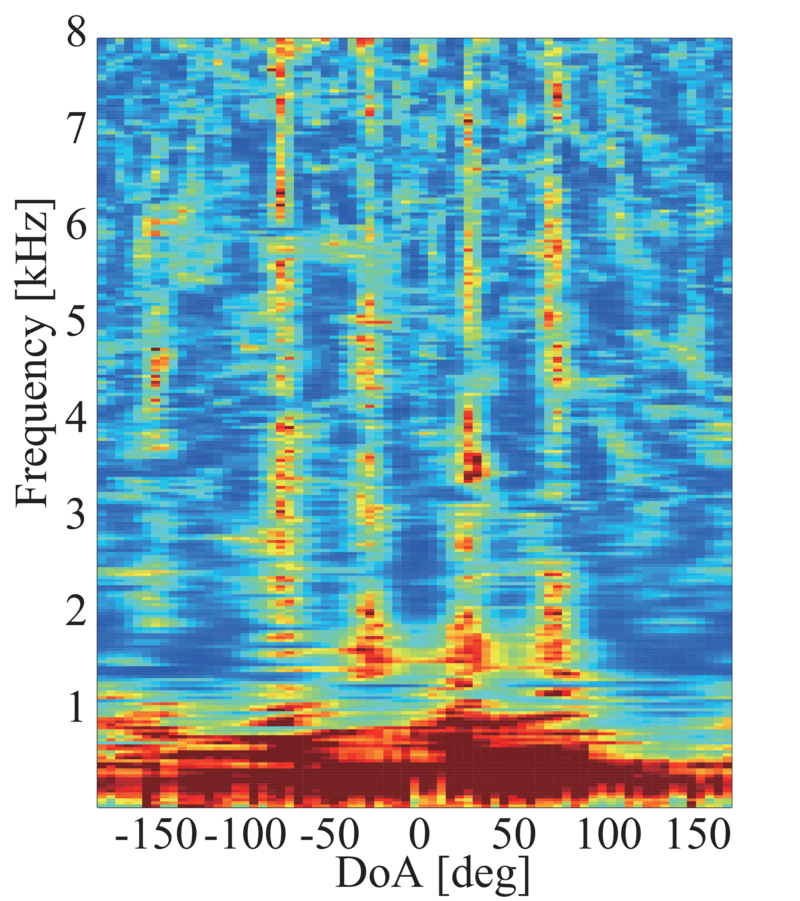(a) MUSIC spectrum when MUSIC_ALGORITHM=SEVD (four speakers)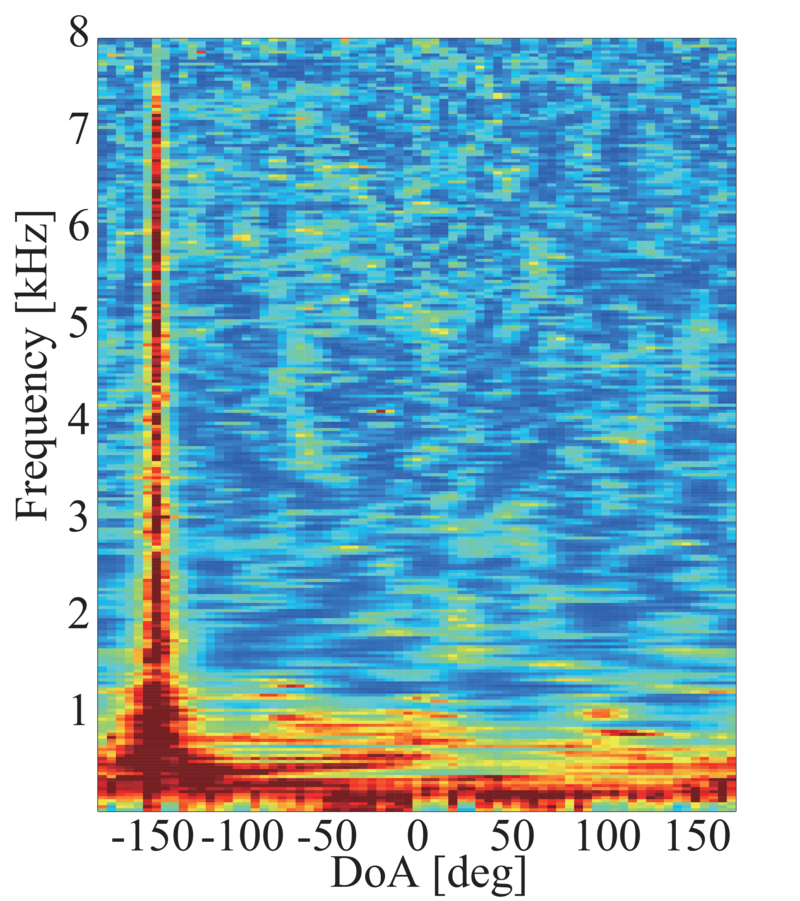(b) MUSIC spectrum of the noise by generating ${\boldsymbol K}(\omega ,f)$ (zero speaker)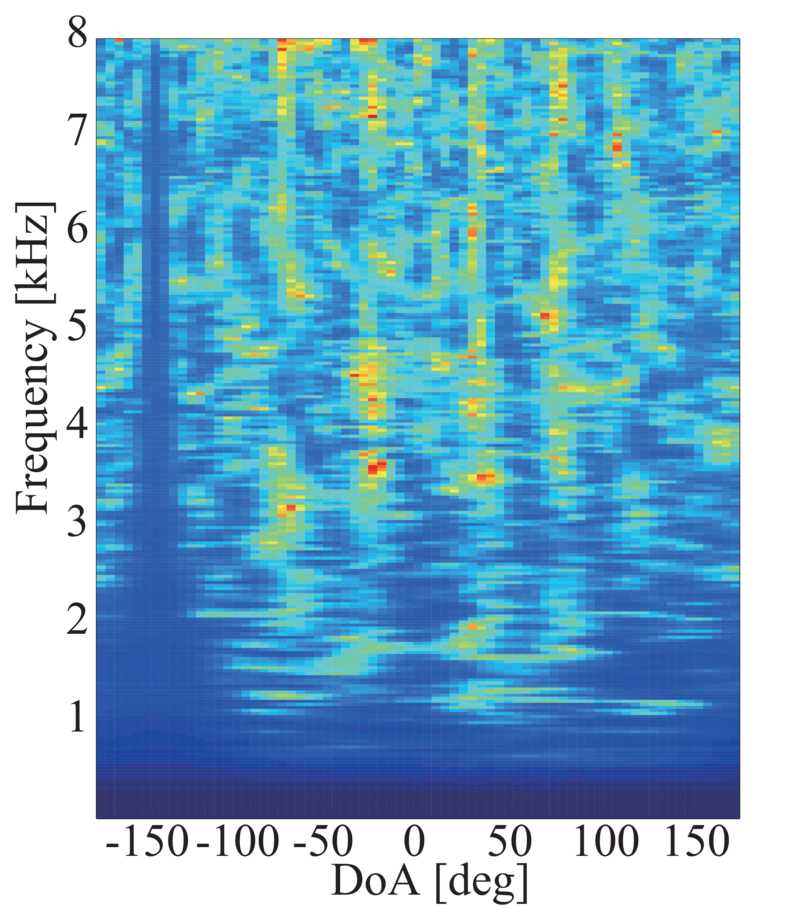(c) MUSIC spectrum when MUSIC_ALGORITHM=GSVD (four speakers)
Figure 6.35: Comparison of MUSIC spectrum

### 6.2.14.6 References

• F. Asano et. al, “Real-Time Sound Source Localization and Separation System and Its Application to Automatic Speech Recognition” Proc. of International Conference on Speech Processing (Eurospeech 2001), pp.1013–1016, 2001.

• Toshiro Oga, Yutaka Kaneda, Yoshio Yamazaki, "Acoustic system and digital processing" The Institute of Electronics, Information and Communication Engineers.

• K. Nakamura, K. Nakadai, F. Asano, Y. Hasegawa, and H. Tsujino, “Intelligent Sound Source Localization for Dynamic Environments”, in Proc. of IEEE/RSJ Int’l Conf. on Intelligent Robots and Systems (IROS 2009), pp. 664–669, 2009.